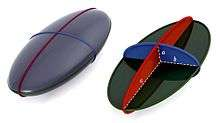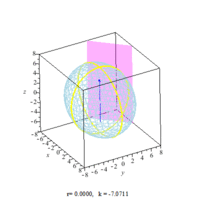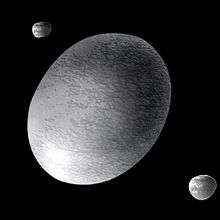# EllipsoidTri-axial ellipsoid with distinct semi-axis lengthsTri-axial ellipsoid with distinct semi-axes a, b and cEllipsoids of revolution (spheroid) with a pair of equal semi-axes (a) and a distinct third semi-axis (c) which is an axis of symmetry. The ellipsoid is prolate (top) or oblate (bottom) as c is greater than or less than a.Circular sections of an ellipsoid

An ellipsoid is a closed quadric surface that is a three-dimensional analogue of an ellipse. The standard equation of an ellipsoid centered at the origin of a Cartesian coordinate system and aligned with the axes isThe points (a,0,0), (0,b,0) and (0,0,c) lie on the surface and the line segments from the origin to these points are called the semi-principal axes of length a, b, c. They correspond to the semi-major axis and semi-minor axis of the appropriate ellipses.

One can assume without loss of generality that. If all three semi-axis are different, then it is a generic tri-axial or (rarely) scalene ellipsoid. If two or three semi-axis are equal (parameter degeneracy), then the ellipsoid is an 'ellipsoid of revolution', which is also called a spheroid. More specifically:

•oblate ellipsoid of revolution (oblate spheroid);
•prolate ellipsoid of revolution (prolate spheroid);
•sphere.

Mathematical literature often uses 'ellipsoid' in place of 'tri-axial ellipsoid'. Scientific literature (particularly geodesy) often uses 'ellipsoid' in place of 'ellipsoid of revolution' and only applies the adjective 'tri-axial' when treating the general case.

Any planar cross section of an ellipsoid forms an ellipse on its surface: this degenerates to a circle for sections normal to the symmetry axis of an ellipsoid of revolution (or all sections when the ellipsoid degenerates to a sphere.)

## Ellipsoid equations

### Cartesian coordinates

#### Parameterization

The ellipsoid may be parameterized in several ways. One possible choice which singles out the 'z'-axis is:whereThe parameters may be interpreted as spherical coordinates. For constant u, that is on the ellipse which is the intercept with a constant z plane, v then plays the role of the eccentric anomaly for that ellipse. For constant v on a plane through the Oz axis the parameter u plays the same role for the ellipse of intersection. Two other similar parameterizations are possible, each with their own interpretations. Only on an ellipse of revolution can a unique definition of reduced latitude be made.

#### Canonical form#### Generalised form

More generally, an arbitrarily oriented ellipsoid, centered at v, is defined by the solutions x to the equationwhere A is a positive definite matrix and x, v are vectors.

The eigenvectors of A define the principal axes of the ellipsoid and the eigenvalues of A are the reciprocals of the squares of the semi-axes:,and. An invertible linear transformation applied to a sphere produces an ellipsoid, which can be brought into the above standard form by a suitable rotation, a consequence of the polar decomposition (also, see spectral theorem). If the linear transformation is represented by a symmetric 3-by-3 matrix, then the eigenvectors of the matrix are orthogonal (due to the spectral theorem) and represent the directions of the axes of the ellipsoid: the lengths of the semiaxes are given by the eigenvalues. The singular value decomposition and polar decomposition are matrix decompositions closely related to these geometric observations.

### Spherical coordinates### Cylindrical coordinates## Volume and surface area

### Volume

The volume bounded by the ellipsoid isNote that this equation reduces to that of the volume of a sphere when all three elliptic radii are equal, and to that of an oblate or prolate spheroid when two of them are equal.

The volume of an ellipsoid isthe volume of a circumscribed elliptic cylinder, andthe volume of the circumscribed box.

The volumes of the inscribed and circumscribed boxes are respectively:### Surface area

The surface area of a general (tri-axial) ellipsoid iswhereand where F(φ,k) and E(φ,k) are incomplete elliptic integrals of the first and second kind respectively.

The surface area of an ellipsoid of revolution (or spheroid) may be expressed in terms of elementary functions:which, as follows from basic trigonometric identities, are equivalent expressions (i.e. the formula forcan be used to calculate the surface area of a prolate ellipsoid and vice versa). In both cases e may again be identified as the eccentricity of the ellipse formed by the cross section through the symmetry axis. (See ellipse). Derivations of these results may be found in standard sources, for example Mathworld.

#### Approximate formulaHere p ≈ 1.6075 yields a relative error of at most 1.061%; a value of p = 8/5 = 1.6 is optimal for nearly spherical ellipsoids, with a relative error of at most 1.178%.

In the "flat" limit of c much smaller than a, b, the area is approximately 2πab.

## Applications

The ellipsoidal shape finds many practical applications:

Geodesy
• Earth ellipsoid, a mathematical figure approximating the shape of the Earth.
• Reference ellipsoid, a mathematical figure approximating the shape planetary bodies in general.
Mechanics
Crystallography
• Index ellipsoid, a diagram of an ellipsoid that depicts the orientation and relative magnitude of refractive indices in a crystal.
• Thermal ellipsoid, ellipsoids used in crystallography to indicate the magnitudes and directions of the thermal vibration of atoms in crystal structures.
Lighting

### Dynamical properties

The mass of an ellipsoid of uniform density ρ is:The moments of inertia of an ellipsoid of uniform density are:For a=b=c these moments of inertia reduce to those for a sphere of uniform density.Artist's conception of Haumea, a Jacobi-ellipsoid dwarf planet, with its two moons

Ellipsoids and cuboids rotate stably along their major or minor axes, but not along their median axis. This can be seen experimentally by throwing an eraser with some spin. In addition, moment of inertia considerations mean that rotation along the major axis is more easily perturbed than rotation along the minor axis.

One practical effect of this is that scalene astronomical bodies such as Haumea generally rotate along their minor axes (as does Earth, which is merely oblate); in addition, because of tidal locking, moons in synchronous orbit such as Mimas orbit with their major axis aligned radially to their planet.

A relaxed ellipsoid, that is, one in hydrostatic equilibrium, has an oblateness a − c directly proportional to its mean density and mean radius. Ellipsoids with a differentiated interior—that is, a denser core than mantle—have a lower oblateness than a homogeneous body. Over all, the ratio (bc)/(ac) is approximately 0.25, though this drops for rapidly rotating bodies.

The terminology typically used for bodies physically rotating on their minor axis -- not necessarily ellipsoids of revolution, in their mathematical construction -- and whose shape is determined by their gravitational field is Maclaurin spheroid (oblate spheroid) and Jacobi ellipsoid (scalene ellipsoid). At faster rotations, piriform or oviform shapes can be expected, but these are not stable.

### Fluid properties

The ellipsoid is the most general shape for which it has been possible to calculate the creeping flow of fluid around the solid shape. The calculations include the force required to translate through a fluid and to rotate within it. Applications include determining the size and shape of large molecules, the sinking rate of small particles, and the swimming abilities of microorganisms.

### In probability and statistics

The elliptical distributions, which generalize the multivariate normal distribution and are used in finance, can be defined in terms of their density functions. When they exist, the density functions f have the structure:whereis a scale factor,is an-dimensional random row vector with median vector(which is also the mean vector if the latter exists),is a positive definite matrix which is proportional to the covariance matrix if the latter exists, andis a function mapping from the non-negative reals to the non-negative reals giving a finite area under the curve. The multivariate normal distribution is the special case in whichfor quadratic form.

Thus the density function is a scalar-to-scalar transformation of a quadric expression. Moreover, the equation for any iso-density surface states that the quadric expression equals some constant specific to that value of the density, and the iso-density surface is an ellipsoid.

## In higher dimensions

The volume of a higher-dimensional ellipsoid (a hyperellipsoid) can be calculated using the dimensional constant given for the volume of a hypersphere. One can also define hyperellipsoids as the images of spheres under invertible linear transformations. The spectral theorem can again be used to obtain a standard equation akin to the one given above.Wikimedia Commons has media related to Ellipsoids.